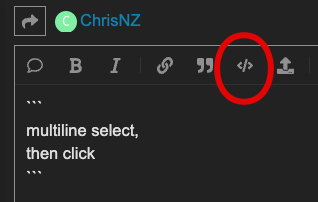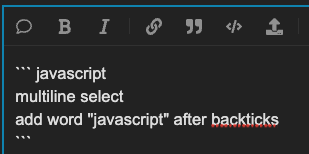# Invalid function call?

secondsPerMode = 8
xFadePct = 2// Percentage of the time we spend in crossfades

modeCount = 1
beforeRenders = array(modeCount)
renderers = array(modeCount)

pinMode(26, OUTPUT)

export function render(delta) {

}

export function beforeRender(delta) {
if(clockHour() < 21 || clockHour() >= 23){
digitalWrite(26,LOW)
}else{
digitalWrite(26,HIGH)
}
modeTime = time(secondsPerMode * modeCount/ 65.536) * modeCount

// 0 when not crossfading; 0…0.999 when crossfading
pctIntoXfFade = max(((modeTime % 1) - (1 - xFadePct)) / xFadePct, 0)

for (var m = 0; m < modeCount; m++) {
beforeRendersm // computes ALL beforeRenders
}
// ToDo: For many patterns combined, enhance that to just call the 2 needed
}

export function render2D(i, x, y) {
/*
If we’re crossfading mode 1 to mode 2, we randomly pick that this pixel will
come from either mode 1’s renderer or mode 2’s. Which one it comes from
is probabilistically related to the percentage we’re into this crossfade.
*/
skew = random(1) < wave((pctIntoXfFade - 0.5) / 2) // wave makes it “tween”

thisPixelMode = floor((modeTime + skew) % modeCount)
renderers[thisPixelMode](i, x, y)
}

// fireworks Dust___________________________________________________________________
//m number between 0 and 100 is less than 90 (i.e. most of the

/*
If a random decimal between 0 and 1 is over 0.995, then the value is 1 and
the pixel is on. Otherwise it’s zero (off). Another way of thinking about
this: The odds of a pixel being on are ~ 5-in-1000, or 1-in-200.
*/

// fast pulse 2D___________________________________________________________________

// house lights R W B_______________(0,0,0)

// Spark Center ___________________________________________________________________
numSparks = floor(pixelCount / 2)

// Middle index of the strip
midIndex = pixelCount / 2

// These constants set the bounds for a newly spawned spark’s initial velocity
ISEM = 0.44 // ISEM = Initial Spark Energy Minimum
ISER = 0.66 * ISEM // ISER = Initial Spark Energy Range

// Each spark has half the strip distance to cover, so halve the friction
friction = 1 / pixelCount / 2
sparks = array(numSparks) // Energy of each spark
sparkX = array(numSparks) // Positions of each sprak
pixels = array(pixelCount) // Heat / intensity in each pixel

for (i = 0; i < numSparks; i++) {
// Initialize each spark’s position to a random point on the strip
sparkX[i] = random(pixelCount)
// Sparks further from the center are older and have less energy
sparks[i] = ISEM * (1 - abs(sparkX[i] - midIndex) / midIndex) + random(ISER)
// Set the sparks left of center to head left (I…E. have negative energy)
if (sparkX[i] < pixelCount / 2) sparks[i] *= -1
}

beforeRenders = function (delta) {
delta *= .1

for (i = 0; i < pixelCount; i++)
pixels[i] *= min(0.1 / delta, 0.99)

// Examining each spark…
for (i = 0; i < numSparks; i++) {
// If a spark has fizzled out…
if (abs(sparks[i]) < 0.001) {
// Set this spark’s energy to a value between ISEM and (ISEM + ISER)
sparks[i] = ISEM + random(ISER)
// Randomly set half the sparks to go left (IE negative energy)
if (random(1) > 0.5) sparks[i] *= -1
// Set the spark’s position back to the center
sparkX[i] = midIndex
}

``````// Slow it down (lose some energy) with friction; preserve the sign
sparks[i] -= friction * delta * (sparks[i] > 0 ? 1 : -1)

/*
Advance the position of each spark proportional to how much time has
passed. sparks[i] is signed, so negative energy reduces its position.
Notice we opted to not use the square of sparks[i] this time. You can,
but don't forget to add the sign back:

sparkX[i] += pow(sparks[i], 2) * delta * (sparks[i] > 0 ? 1 : -1)

As-is, it functions more like a velocity, where Δx = v ⋅ Δt
*/
sparkX[i] += sparks[i] * delta

/*
If a spark's position exceeds either end of the strip, reset its energy
to 0. It'll be reinitialized in the next beforeRender(). The position in
sparkX also needs to be set to zero in order to avoid an "array index out
of bounds" error in the next part.
*/
if (sparkX[i] >= pixelCount || sparkX[i] < 0) {
sparkX[i] = 0
sparks[i] = 0
}

// Since negative energy is allowed, we need to drop the sign when
// accumulating the heat
pixels[sparkX[i]] += abs(sparks[i])
``````

}
}

renderers = function (index, x, y) {
v = pixels[xpixelCount] // Brightness is the heat in this pixel
v
v*v // Gamma correction. Small v (0 < v < 1) becomes smaller.

/*
h: The hue is set to 0.63, so sparks cool (saturate) to blue
s: Make hot/fast pixels white (v close to or above 1 means s is near 0)
v: The heat (brightness) in the pixel
*/
randomColorIndex = round(random(11))
if(x < .40){ // RED
hsv24(1, 2 - v, v)
}
else if(x < .60){ // WHITE
hsv24(0, 0, v)
}else{ // BLUE
hsv24(.63, 2 - v, v)
}
/if(randomColorIndex <= 5){
hsv24(1, 2 - v, v)
}
else if(randomColorIndex <= 10){
hsv24(.63, 2 - v, v)
}else{
hsv24(0, 0, v)
}
/
}

I was hoping somebody could help me out with this error I’m sure it’s something simple. Thank you,
Twilight
Sorry about the format was trying to upload just the copy of the pattern.

Did you forget brackets in your call ro beforeRenders?

That’s what it was
Thank You!

1 Like

Hey @Twilight! If you’d like to get your code to display nicely on the forums, you can use the code block formatting in markdown.

Here’s how to do it with examples

Cheers!

On this note, is there any chance a link to some simple documentation on markdown syntax (with examples) can be added to the edit box via a “help” icon or something? There doesn’t appear to be anything currently, or if it’s there it’s not obvious enough for me to see. I imagine it’ll be very useful for first time users as well as occasional ones like me who’re not too familiar with the syntax - I often have to Google to refresh my memory on how to format code and so on. A “code” icon would be very useful too…

I agree! Technically that’s what this html tag icon (hover: “preformated text”) does in the editor when you select multiple lines, but you have to remember to add code highlighting by adding the word “javascript”.Better:Thank you! I couldn’t figure out how to just upload the file to The Forum.

Twilight